# The ‘Vulcan’ strategy. Is it profitable or not?

### Intro

Hi there and welcome to this blog series where I will be searching for the best trading strategy for cryptocurrency trading. In each blogpost/video I am going to test a strategy that is explained in a YouTube video, trading site or is publicly available for use.  Each strategy will be tested on its performance by trading multiple digital asset pairs over their largest possible backtest period. The result of this test is then compared with the results of earlier tested strategies.

If you like these articles and you want to see how I execute these tests, then see the video that I included in this post.

Please remember that this post is for entertainment and educational purposes only. Everything you see is hypothetical and based on historic data so there’s no guarantee the investigated strategy will work in the future. I am also not a professional, so always do your own research or consult a professional before using any information in real trading.

In this post I am going to test a strategy that is called the “Vulcan” strategy. I have no clue why it is called the “Vulcan”, but then again I have heard stranger names for a strategy. I found this strategy a while ago on the Internet and have forgotten the original author. Unfortunately his or her name is also not in the strategy. So if you recognize this strategy, then all credits go to you.

### The setup

See this blog post to know more about the approach I use when making these backtests: https://www.publish0x.com/dutchcryptodad/is-it-profitable-or-not-the-setup-and-approach-xppgjoj

### Strategy

Let’s take a look at the code to see whats going on: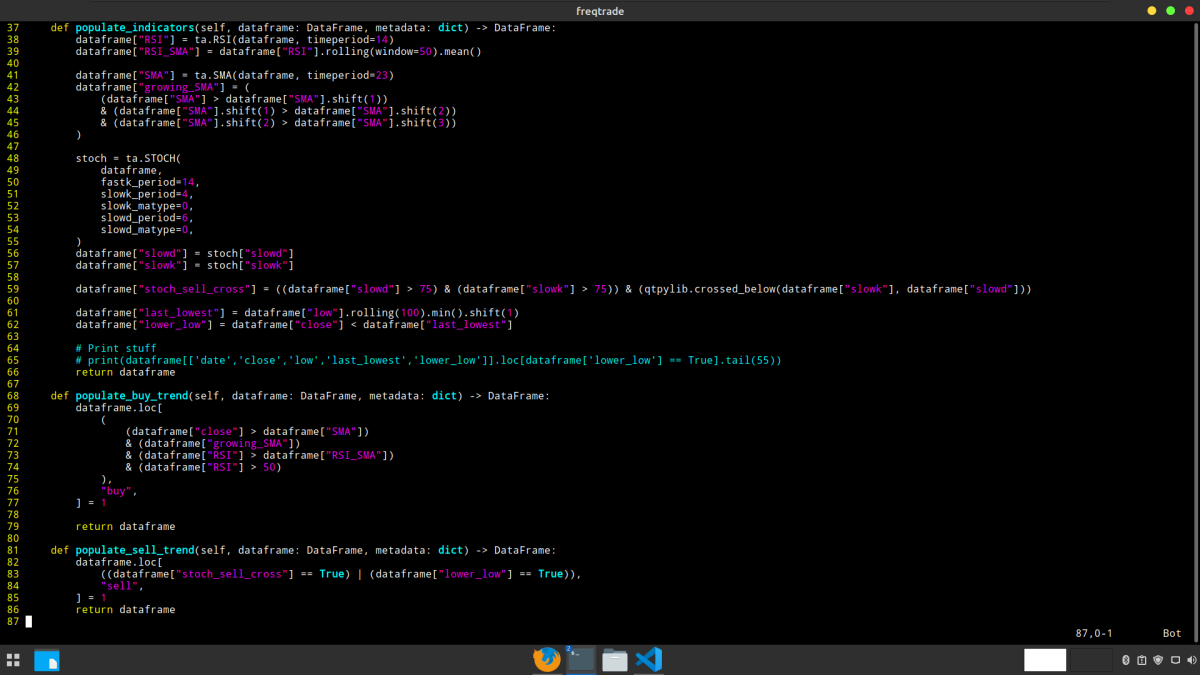I see that the original timeframe of this code is the 30 minute timeframe.

The author has configured a stop loss of 25% to let the trade breathe I think

The takeprofit point in the form of the ROI has not been set. I see this because it is configured to 100. So the exit signals come from the indicators that are configured in the code.

What I can see from the code is that there is a mix of indicators and custom triggers.

The base indicators used here are the Simple moving average, RSI and stochastics indicator. And if you want to know more about these indicators I suggest you read up on the on the internet.

The RSI also has a 50 day moving average added.

The stochastics slowk period and slowd period are not the default ones but are custom. On the chart I see that these changes cause the slowk and slowd not to cross each other this much as with the standard values.

An interesting thing here is the “growing sma” parameter. Apparently the author uses a true value here if the closeprice of yesterday is higher than the day before and the close of the day before is higher than the day before that. Which means that the SMA is rising over the previous three days. Which is very ingenious.

There is also a custom trigger called stoch_sell_cross configured that gives a true value if the slowd and slowk value are above 75 and then the slowk crosses below the slowd.

Also there is another exit signal configured that uses a saved lowest low candle value over the last 100 candles and when price comes below that value, then an exit signal is given.

You can also see this in the buy and sell trend functions further down the code

The buy signal is given when

• Closeprice is above the simple moving average
• The growing sma value is equal to true, so there must be an rising sma
• The rsi is higher than the rsi sma and finally the rsi should be above the 50 level

SELL signal

In this strategy there are only sell signals if stop loss gets hit or when the following triggers happen

You can see that the stoch_sell_cross can give a sell signal so when slowd and slowk are above 75 and a bearish cross occurs then a sell signal happens.

But also when the closeprice gets below the last lowest price over the last 100 days according to the configured trigger.

I assume this last one acts like a moving stop loss but also as an exit signal when the price gets under that last lowest price.

So if I use these on a chart it looks like this:

### Initial backtest results

According to the backtest over all the available timeperiods I have the following results: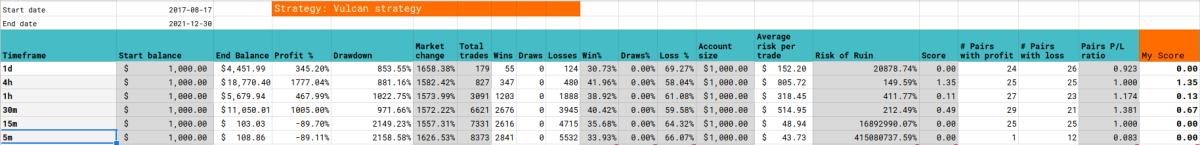• Best timeframe: 4 hour
• Total profit of strategy: 1777 %
• Drawdown of strategy: 881 %
• Winrate 42 %
• Risk of ruin of strategy: 150 %

It’s nice to see that a strategy that was made for a lower timeframe also actually performs better on that lower timeframe than the daily timeframe. However the 4 hour still seems to give the best odds of having a profitable strategy. So I build further on that timeframe.

The only thing I do not really like is the drawdown of 881 percent. The other values seem to be quite reasonable.

And come to think about it, all those custom measures to try to get winning trades or prevent losing trades did not seem to pay off that much. The winrate is still moderate as you can see from the backtest results.

### Hyperparameter optimisation

I have created a hyperopt file from this strategy to see if I can get even better results from this strategy. My initial thought is that the buy triggers primarily come from the simple moving average and the rsi level. The sell trigger primarily comes from the horizontal line where slowd and slok must be above and cross to trigger a sell signal.

So what I’ve done is add three spaces to find the optimal sma, rsi level, and the stochastics level. I did not touch other indicators or parameters. Maybe I could have taken these with the optimisation as well but I did not want to push it too far and have the risk of curve fitting too much.

The optimal rsi level and stochastics level are determined in the buy and sell functions.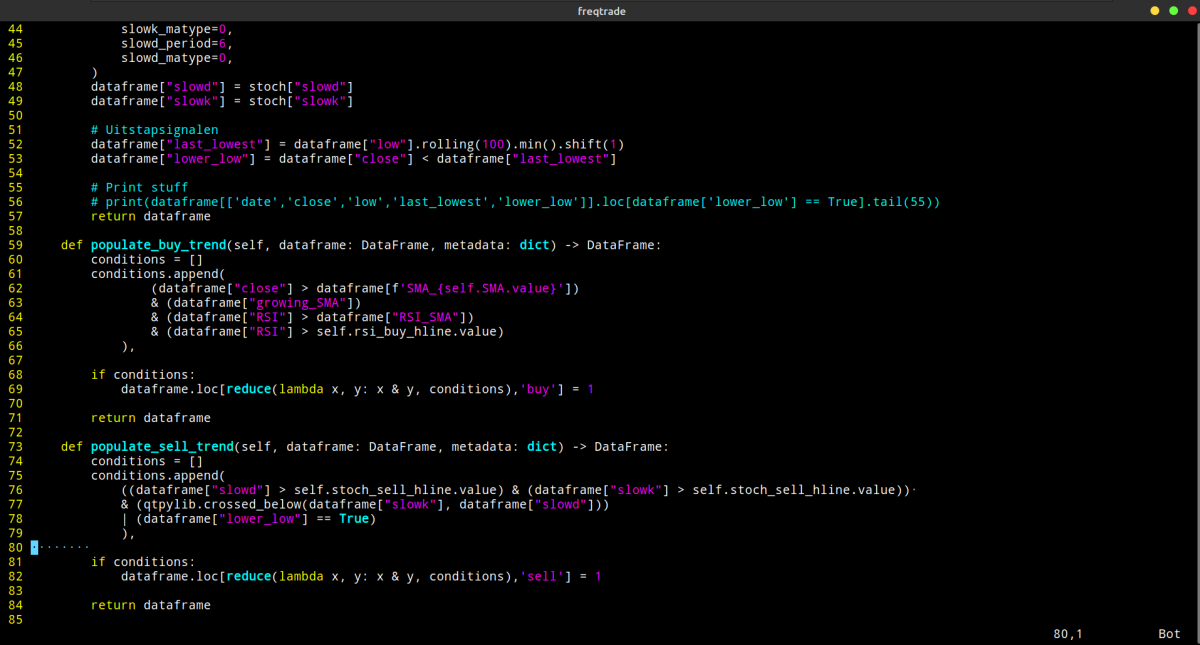So now that I have done this, let's see if I can use Hyperparemeter optimization to improve this strategy.

### Conclusions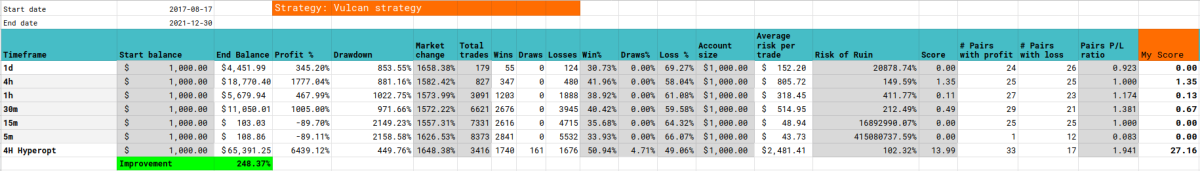After optimizing again I see some very nice improvements. The hypothetical gains were optimized by almost 250 percent. And again this comes primarily from setting the ROI takeprofit levels and not as much from the sell signals. Also the drawdown significantly improved by 50 percent and I assume that’s from taking profits on the right time.

The sell signal still has a lot losses and I am wondering if this can be avoided by some other indicator that only allows this strategy to trade under bullish circumstances. Like for example a supertrend indicator. But that is for another time to find out maybe.

Also do not assume that if you copied this approach 100% that you should have the same results. That's because of the way hyperopt works and you can learn this in my Hyperparameter optimization video.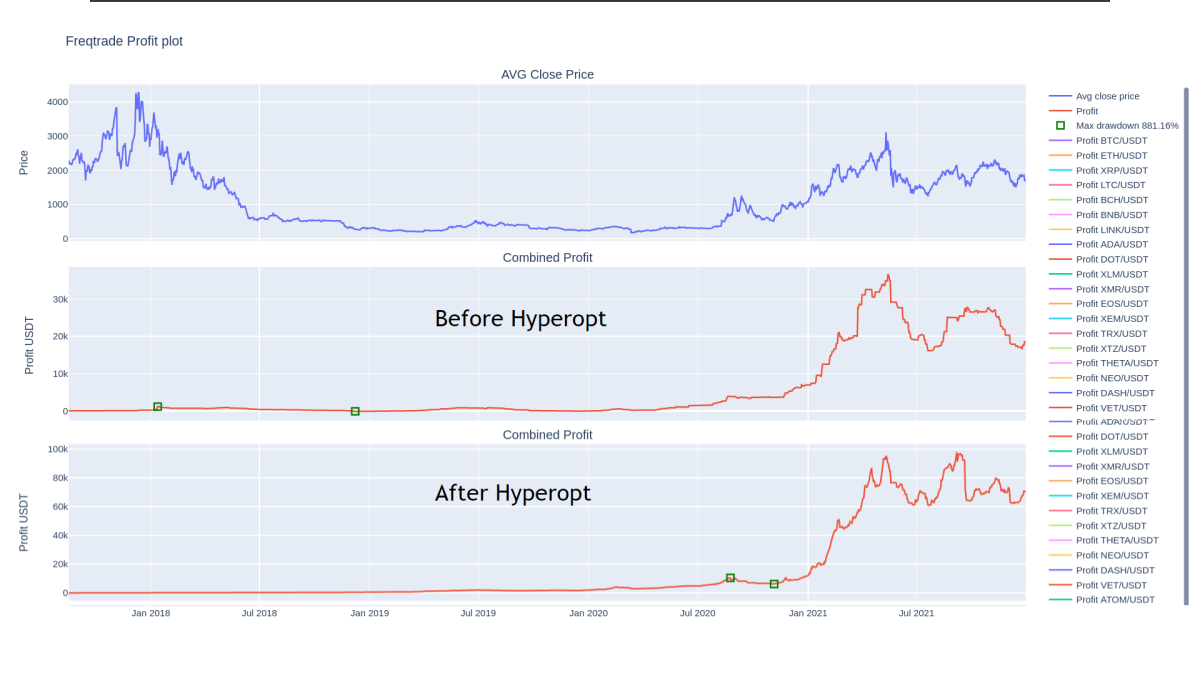If I compare the before hyperopt and after hyperopt profit chart then I immediately see a big difference in the period the drawdown occurred. In the original strategy this drawdown was a slow long bleed of funds from what i think is the end of the previous bull market to the begin of the current bull market. The drawdown from the hyperopted strategy is much shorter and also less a burden on the hypothetical funds.

The most current timeperiods also show the difference in the two trading configurations. The pre hyperopt graph has the highest peak of around 40000 usdt but the hyperopted graph stops around 100 k and never goes below 60k again.  There is no period where there is no trade because there is no flat line. So in these bearish periods the bot still trades and that’s where an extra indicator may come in handy to filter out these bearish periods. But as said earlier that's for an enthusiastic reader to find out.

### Strategy League

The list get’s nice and long and this is already the 11th strategy that I’m testing.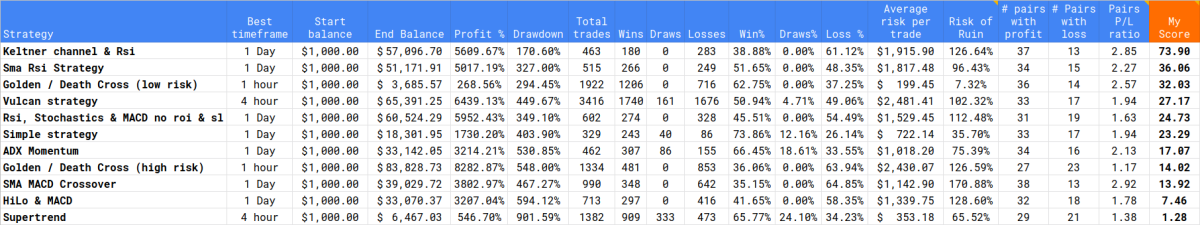The Vulcan strategy enters the overall league in the 4th position.

It has the highest hypothetical profits in the top5 and the winrate of 50% is better than the number 1 in the list.

Also the risk of ruin is better than the numbers 2 and three.

The only drawback is the relatively high drawdown that this strategy has.

But as I said earlier, I think that an extra bullish/bearish market condition indicator can easily filter out bad trades under bearish conditions.

The relative high amount of trades will cost you trading fees so also be aware of this.

### The strategy code

``````# --- Do not remove these libs ---
from pandas import DataFrame

import talib.abstract as ta
import pandas_ta as pta
import numpy as np  # noqa
import pandas as pd  # noqa

# These libs are for hyperopt
from functools import reduce
from freqtrade.strategy import (BooleanParameter, CategoricalParameter, DecimalParameter,IStrategy, IntParameter)

class vulcan(IStrategy):
stoploss = -0.25
timeframe = "30m"
minimal_roi = {"0":  100}

plot_config = {
"main_plot": {
"SMA": {"color": "red"},
},
"subplots": {
"STOCH": {
"slowd": {"color": "blue"},
"slowk": {"color": "orange"},
},
"RSI": {
"RSI": {"color": "blue"},
"RSI_SMA": {"color": "orange"},
},
},
}

def populate_indicators(self, dataframe: DataFrame, metadata: dict) -> DataFrame:
dataframe["RSI"] = ta.RSI(dataframe, timeperiod=14)
dataframe["RSI_SMA"] = dataframe["RSI"].rolling(window=50).mean()

dataframe["SMA"] = ta.SMA(dataframe, timeperiod=23)
dataframe["growing_SMA"] = (
(dataframe["SMA"] > dataframe["SMA"].shift(1))
& (dataframe["SMA"].shift(1) > dataframe["SMA"].shift(2))
& (dataframe["SMA"].shift(2) > dataframe["SMA"].shift(3))
)

stoch = ta.STOCH(
dataframe,
fastk_period=14,
slowk_period=4,
slowk_matype=0,
slowd_period=6,
slowd_matype=0,
)
dataframe["slowd"] = stoch["slowd"]
dataframe["slowk"] = stoch["slowk"]

dataframe["stoch_sell_cross"] = ((dataframe["slowd"] > 75) & (dataframe["slowk"] > 75)) & (qtpylib.crossed_below(dataframe["slowk"], dataframe["slowd"]))

dataframe["last_lowest"] = dataframe["low"].rolling(100).min().shift(1)
dataframe["lower_low"] = dataframe["close"] < dataframe["last_lowest"]

# Print stuff
# print(dataframe[['date','close','low','last_lowest','lower_low']].loc[dataframe['lower_low'] == True].tail(55))
return dataframe

dataframe.loc[
(
(dataframe["close"] > dataframe["SMA"])
& (dataframe["growing_SMA"])
& (dataframe["RSI"] > dataframe["RSI_SMA"])
& (dataframe["RSI"] > 50)
),
] = 1

return dataframe

def populate_sell_trend(self, dataframe: DataFrame, metadata: dict) -> DataFrame:
dataframe.loc[
((dataframe["stoch_sell_cross"] == True) | (dataframe["lower_low"] == True)),
"sell",
] = 1
return dataframe
``````

### The hyperopt code

``````# --- Do not remove these libs ---
from pandas import DataFrame

import talib.abstract as ta
import pandas_ta as pta
import numpy as np  # noqa
import pandas as pd  # noqa

# These libs are for hyperopt
from functools import reduce
from freqtrade.strategy import (BooleanParameter, CategoricalParameter, DecimalParameter,IStrategy, IntParameter)

class vulcanhopt(IStrategy):
stoploss = -0.25
timeframe = "30m"
minimal_roi = {"0":  100}

# Hyperopt spaces
SMA = IntParameter(13, 56, default=21, space="buy")
stoch_sell_hline = IntParameter(65, 85, default=75, space="sell")

def populate_indicators(self, dataframe: DataFrame, metadata: dict) -> DataFrame:
# Instapsignalen
dataframe["RSI"] = ta.RSI(dataframe, timeperiod=14)
dataframe["RSI_SMA"] = dataframe["RSI"].rolling(window=50).mean()

for val in self.SMA.range:
dataframe[f'SMA_{val}'] = ta.SMA(dataframe, timeperiod=val)
# dataframe["SMA"] = ta.SMA(dataframe, timeperiod=23)
dataframe["growing_SMA"] = (
(dataframe[f'SMA_{val}'] > dataframe[f'SMA_{val}'].shift(1))
& (dataframe[f'SMA_{val}'].shift(1) > dataframe[f'SMA_{val}'].shift(2))
& (dataframe[f'SMA_{val}'].shift(2) > dataframe[f'SMA_{val}'].shift(3))
)

stoch = ta.STOCH(
dataframe,
fastk_period=14,
slowk_period=4,
slowk_matype=0,
slowd_period=6,
slowd_matype=0,
)
dataframe["slowd"] = stoch["slowd"]
dataframe["slowk"] = stoch["slowk"]

# Uitstapsignalen
dataframe["last_lowest"] = dataframe["low"].rolling(100).min().shift(1)
dataframe["lower_low"] = dataframe["close"] < dataframe["last_lowest"]

# Print stuff
# print(dataframe[['date','close','low','last_lowest','lower_low']].loc[dataframe['lower_low'] == True].tail(55))
return dataframe

conditions = []
conditions.append(
(dataframe["close"] > dataframe[f'SMA_{self.SMA.value}'])
& (dataframe["growing_SMA"])
& (dataframe["RSI"] > dataframe["RSI_SMA"])
),

if conditions:
dataframe.loc[reduce(lambda x, y: x & y, conditions),'buy'] = 1

return dataframe

def populate_sell_trend(self, dataframe: DataFrame, metadata: dict) -> DataFrame:
conditions = []
conditions.append(
((dataframe["slowd"] > self.stoch_sell_hline.value) & (dataframe["slowk"] > self.stoch_sell_hline.value))
& (qtpylib.crossed_below(dataframe["slowk"], dataframe["slowd"]))
| (dataframe["lower_low"] == True)
),

if conditions:
dataframe.loc[reduce(lambda x, y: x & y, conditions),'sell'] = 1

return dataframe``````

### The hyperopt json results

``````{
"strategy_name": "vulcanhopt",
"params": {
"trailing": {
"trailing_stop": false,
"trailing_stop_positive": null,
"trailing_stop_positive_offset": 0.0,
"trailing_only_offset_is_reached": false
},
"SMA": 35,
},
"sell": {
"stoch_sell_hline": 65
},
"protection": {},
"roi": {
"0": 0.158,
"726": 0.111,
"1321": 0.081,
"6392": 0
},
"stoploss": {
"stoploss": -0.323
}
},
"ft_stratparam_v": 1,
"export_time": "2022-02-14 11:57:32.793352+00:00"
``````I'm just a regular Dutch dad with a passion for crypto, trading, technology and learning. This channel is my personal journey into the world of Crypto, blockchain, programming, trading bots, trading strategies, NFT's, Defi and many things more.